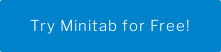Generally speaking, if you are a chemical engineer, you are probably developing and designing chemical manufacturing processes. Unlike other engineers, that means you may need to apply the principles of chemistry, biology, physics, and math to solve problems that involve the production or use of chemicals, fuel, drugs, food, and many other products. With all that time dedicated to the sciences, if you did not spend as much time on statistics as you wish you did, have no fear, Minitab is here! Now, let's talk about why Analysis of Variance (ANOVA) can be a chemical engineer's secret weapon.

## Why You Should Know ANOVA

Many industrial applications involve experiments where the goal is the understand if groups are different. In statistical terms, we consider a factor- let us say catalyst type- and we want to understand if the levels of that factor- let us say catalyst 1, catalyst 2, catalyst 3 and catalyst 4 - are statistically significantly different from one another. When the measurements across the groups are continuous and certain other assumptions are met, we use ANOVA to compare the means of the groups. In a sense, the name "Analysis of Variance" is a misnomer, because we are interested in comparing the means of the groups. However, by analyzing the variation in the data within the group levels and between the groups, we can determine if the group means are statistically different.

### Learn about more visualizations and graphs that can make your insights easy to share and easier to understand!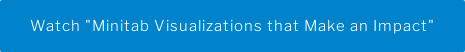ANOVA tests the null hypothesis that the population means (noted with the symbol µ ) are all equal. We will estimate the population means using the sample means. If this null hypothesis is rejected, then we conclude that the population means are not all equal.

The null hypothesis:

Ho: µCatalyst 1 = µCatalyst 2 = µCatalyst 3 = µCatalyst 4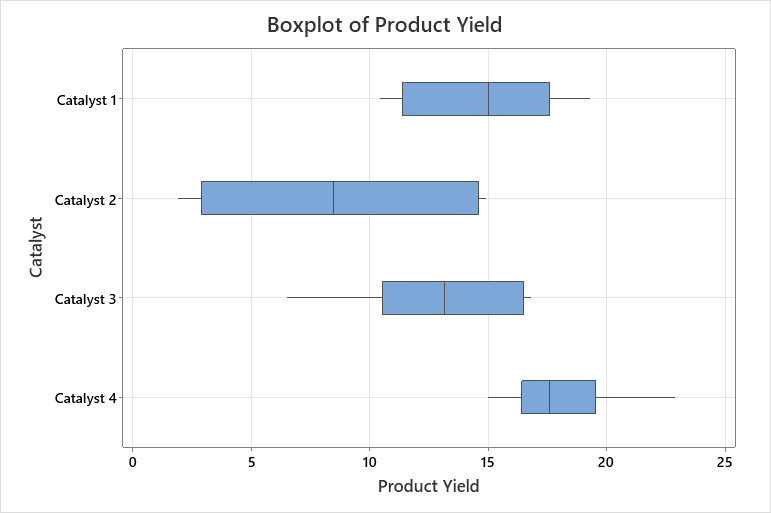In plain language, we assume the means are all equal across the groups, and we gather evidence against that-meaning that if we observe large mean differences between these means, we are more likely to reject that belief and assume there are differences within the group levels.

## EXAMPLE OF A ONE-WAY ANOVA

Imagine that a chemical engineer wants to compare the product yield of using four different catalysts.  She heats up the catalyst with the product to get a reaction. Using ANOVA, the engineer can determine whether the product yield using different catalysts differs significantly.

First, the engineer collects her data, as you can see below.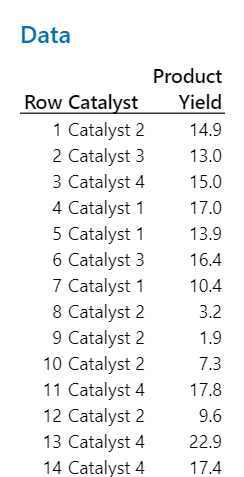Next the engineer runs a one-way ANOVA.

The p-value for the Product Yield ANOVA is small, indicating that there is a very small chance that we would have observed these results if the null hypothesis is true, that is, that the catalyst means are all equal. Because the p-value is less than a 5% significance level (we are using an alpha = 0.05), we reject the null hypothesis. We conclude that t the mean product yield differs across the catalyst groups.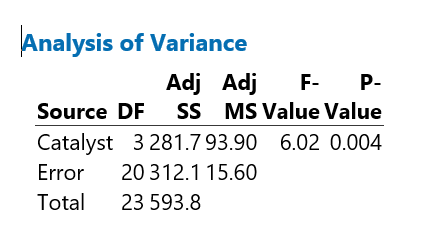The engineer knows that some of the group means are different. The next logical question is, which ones?

## Using Tukey’s Method to conduct the Multiple Comparisons

While ANOVA taught us that the some of the group means are different, the engineer needs a more in-depth comparison to understand which group means are different. Minitab provides “Comparisons” for this very reason. In our example, the chemical engineer uses the Tukey comparisons to formally test for differences between the pairs of groups, to understand which ones are statistically significantly different.

Tukey's multiple comparison test is the most conservative test of several tests that can be used to determine which means amongst a set of means differ from the rest. Tukey's method is used after ANOVA (which is why you may hear the method referred to as a post hoc test) and can be used to create confidence intervals for all pairwise differences between factor level means while controlling the family error rate to a level you specify.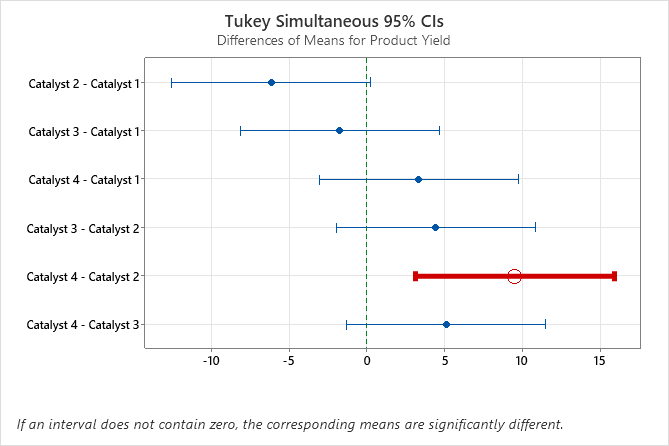In our example, the graph that includes the Tukey simultaneous confidence intervals show that the confidence interval for the difference between the means of Catalyst 2 and 4 is 3.114 to 15.886. This range does not include zero, which indicates that the difference between these means is significant. The engineer can use this estimate of the difference to determine whether the difference is practically significant.

Conversely, the confidence intervals for the remaining pairs of means all include zero, which indicates that the differences are not significant.

## Why not just run a bunch of t-tests to identify differences?

It’s a great question, and one that comes up a lot! The answer has to do with the risk of making a mistake, specifically, the risk of incorrectly concluding there is a statistically significant difference, which is what we call our alpha risk. When we conduct one test, there is a 5% chance we will say there is a difference, when in reality there isn’t. For the 4 catalysts, that would result in 6 t-tests!

What is the probability of observing at least one significant result just due to chance?

P(at least one significant result) = 1 − P(no significant results)

= 1 − (1 − 0.05)6

≈ 0.264

So, with 6 tests being considered, we have a 26% chance of observing at least one significant result, even if all of the tests are actually not significant. Post hoc tests control the experiment-wise error rate; to put it more simply, we want to ensure that the chance we incorrectly declare any pair of catalysts is different remains at 5%. That is exactly what Tukey’s test does for us!

## THE ANSWERS ARE IN ANOVA

Using ANOVA enables a chemical engineer to test mixtures to see if the results are statistically significant. Equally important, she can also determine if the entire group is different or perhaps just a subsegment of the group using comparison tests. In our example, only catalyst 2 and catalyst 4 are statistically significant different regarding product yield. Based on this information, the chemical engineer may begin to look at the other catalysts to determine which catalyst is the most cost-effective, has the longest shelf-life, or is easiest to access knowing that it will yield a similar amount of product.

### Try some comparisons and analysis yourself. Download a free 30-day trial of Minitab Statistical Software today!